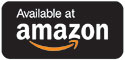Catalog Higher Ed Mathematics Introduction to Linear Algebra: Models, Methods, and Theory

# Introduction to Linear Algebra: Models, Methods, and Theory

## by Alan Tucker

### Print Version

#### Paperback Price: \$35.59

ISBN: 978-1-50669-672-0

540 pages

Suggested retail price shown above.This book provides students with a unified introduction to the models, methods, and theory of modern linear algebra. It introduces students to economic input-output models, population growth models, Markov chains, linear programming, computer graphics, regression and other statistical techniques, and more, which reinforce each other and associated theory.

This book develops linear algebra around matrices. This book puts problem solving and an intuitive treatment of theory first, with a proof-oriented approach intended to come in a second course, in the same way that calculus is taught. The material is unified by the repeated use of a few linear models to illustrate each new concept and technique. These models help students visualize new ideas as they're taught and remember them after the course is complete. Although the book is often informal, it also covers several topics normally left to a more advanced course such as matrix norms, matrix decompositions, and approximation by orthogonal polynomials. This book presents a healthy interplay between mathematics and computer science, that is between continuous and discrete models of thinking.